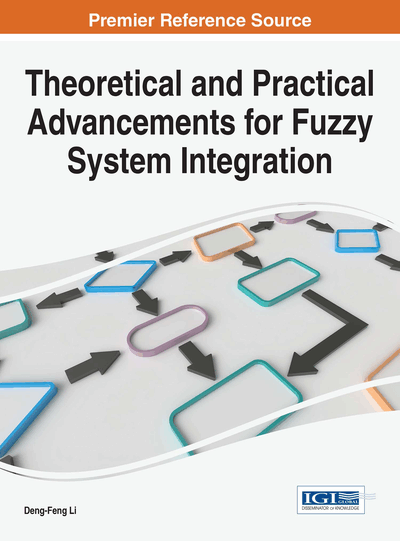# Interval-Valued Intuitionistic Fuzzy Multi-Attribute Decision Making Based on Satisfactory Degree

Gao-Feng Yu (Sanming University, China), Deng-Feng Li (Fuzhou University, China) and Jin-Ming Qiu (Sanming University, China)
DOI: 10.4018/978-1-5225-1848-8.ch003

## Abstract

The aim of this paper is to propose a satisfactory degree method by using nonlinear programming for solving multi-attribute decision making (MADM) problems in which ratings of alternatives on attributes is expressed via interval-valued intuitionistic fuzzy (IVIF) sets and preference information on attributes is incomplete. Concretely, a nonlinear programming model is firstly explored to determine the satisfactory degree which is the ratio of the square of the weight Euclidean distance between an alternative and the IVIF negative ideal solution (IVIFNIS) to the sum of the square of the weight Euclidean distance between the IVIF negative ideal solution (IVIFNIS) and the IVIF positive ideal solution (IVIFPIS). Another nonlinear programming model is also developed to obtain satisfactory intuitionistic fuzzy sets, and then the general satisfactory degrees of the satisfactory intuitionistic fuzzy sets are used to generate the ranking order of the alternatives. Finally, a real example is employed to verify the applicability of the proposed approach and illustrate its practicality and effectiveness.
Chapter Preview
Top

## Introduction

Due to the high complexity of socioeconomic environments, there are some difficulties for a single decision maker (DM) to consider all important aspects of some decision problems in real decision making. Therefore, group decision making under fuzzy environment problem is an interesting research topic and has received more and more attention from researchers during the last several years. A fuzzy multi-attribute decision making (MADM) problem is to choose the best solutions (i.e., alternatives) from all feasible alternatives on multiple attributes by using the fuzzy set introduced by Zadeh (1965). The fuzzy set has been successfully applied to many practical decision making problems. It only uses a membership function which assigns to each element of the universe of discourse a number from the interval [0, 1] in order to indicate the degree of membership to the fuzzy set under consideration. The degree of non-membership is just automatically the complement to 1 of the membership degree to the fuzzy set. However, a human being who expresses the degree of membership of a given element in a fuzzy set very often does not express the corresponding degree of non-membership as the complement to 1. Then Atanassov (1983) introduced the concept of an intuitionistic fuzzy (IF) set that is characterized by two functions which are used to express the degree of membership and the degree of nonmembership, respectively. The IF set seems to be more accurate for uncertainty quantification and provides an opportunity to precisely model the problem based on the existing knowledge and observations. It may not be easy to identify exact value for both the degree of membership and the degree of non-membership of an element to a given set. In this case, a range of values may be a more appropriate measurement to accommodate the uncertainty, imprecision or vagueness.

The interval-valued intuitionistic fuzzy (IVIF) set (Atanassov, 1989), characterized by a membership function and a non-membership function whose values are intervals rather than real numbers, seems to be more suitable for describing the MADM problems in which membership and non-membership degrees of alternatives on attributes cannot be expressed by exact numerical values. In recent years, many scholars discussed the IVIF set' basic operators and their:

• Properties (Chen et al., 2012; Lakshmana et al., 2011),

• Aggregating operators (Xu, 2007; Yu, 2013; Cai, 2014),

• Topological properties (Mondal, 2001,

• Information measures (Ye, 2009; Huang, 2013; Wei, 2011; Xu, 2010; Zhangetal., 2010) and

• Correlation coefcient(Bustince et al., 1995; Park et al., 2009; Wei et al., 2011; Li, 2011),

• Distances (Grzgorzewski, 2004).

## Complete Chapter List

Search this Book:
Reset